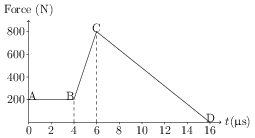# Impulse

## Problems from IIT JEE

Problem (IIT JEE 2002): Two blocks of masses 10 kg and 4 kg are connected by a spring of negligible mass and placed on a frictionless horizontal surface. An impulse gives a velocity of 14 m/s to the heavier block in the direction of the lighter block. The velocity of the centre of mass is,

1. 30 m/s
2. 20 m/s
3. 10 m/s
4. 5 m/s
Consider the block of mass $M={10}\;\mathrm{kg}$, the block of mass $m={4}\;\mathrm{kg}$, and the spring together as a system. The impulse given to the system is, \begin{align} \Delta p=MV=10\times 14={140}\;\mathrm{kg\, m/s}. \end{align} This impulse is equal to the change in linear momentum of the centre of mass of the system i.e., \begin{align} (M+m)V_\text{cm}=140, \end{align} which gives $V_\text{cm}={10}\;\mathrm{m/s}$. Note that the velocity $v$ of the lighter block of mass $m$ immediately after the impulse is zero as, \begin{align} V_\text{cm}=\frac{MV+mv}{M+m}=\frac{(10)(14)+(4)(0)}{10+4}={10}\;\mathrm{m/s}.\nonumber \end{align}

Problem (IIT JEE 1994):The magnitude of the force (in newton) acting on a body varies with time $t$ (in microseconds) as shown in the figure. AB, BC and CD are straight line segments. The magnitude of the total impulse of the force on the body from $t={4}\;\mathrm{\mu s}$ to $t={16}\;\mathrm{\mu s}$ is _______ N s.

Solution: The impulse, $I=\int F\mathrm{d}t$, is the area under the $F\text{-}t$ curve from $t={4}\;\mathrm{\mu s}$ to $16\;\mathrm{\mu s}$. This area is given by, \begin{align} I=(2\times200+\tfrac{1}{2}\times 2\times 600+\tfrac{1}{2}\times 10\times 800){10}^{-6}=5\times{10}^{-3}\;\mathrm{N\,s}.\nonumber \end{align}